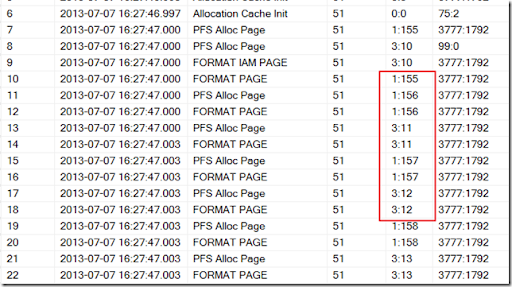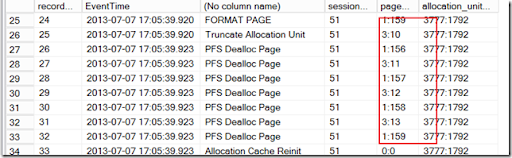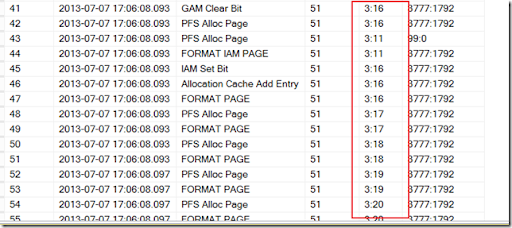## Sunday, July 7, 2013

### 0Tempdb File usage

This post is primary base on the Bob ward’s “Inside tempdb” session in the SQL SUMMIT 2011 .

• Round robin and proportional fill .
• Proportion calculation is base on
• After recovery
• When adding or removing files
• Every 8192 extent allocations

## For extend grow ( Both uniform extend and mixed extend)

• Find the free extend in the current file. ( for uniform extend, looks for GAM, for mix extend, look for SCAM)
• If no space is the current file, find extend in other file.
• If no free space on all files, grow the file

## De allocation

• Both uniform extend and mix extend will need to update PFS.
• Mix extend needs to update SCAM, uniform extend needs to update GAM.
• For tables less than 8 MB, sql server keep one pages and one IAM page.
• For tables larger than 8MB, sql server keeps the system table object.

## Tempdb contention

A lot of allocation and de allocation will cause the temp db contention on GAM, SCAM ,PFS and internal objects.

The symptom is to find the PAGELATCH wait in tempdb files

Solution

• Temp Table caching
• Trace flag 1118; Server wide setting. Except for IAM page, all the pages allocation would use  uniform extend.
• Multiple data files. so the GAM,SCAM can be spread out to multiple files.
• Prevent autogrow. –T 1117 would cause all the file grow the same. It is server wide setting.
• Evaluate temp table usage
• Find out what is consuming internal objects

## Trace flag 1106

Trace flag 1106 can use to see the file switch in the  sys.dm_os_ring_buffers

Create 2 tempdb file and restart the SQL server

`USE [master]`
`GO`
`ALTER DATABASE [tempdb] MODIFY FILE ( NAME = N'tempdev', SIZE = 100Mb)`
`GO`
`ALTER DATABASE [tempdb] `
`ADD FILE ( NAME = N'tempdev2', FILENAME = N'C:\Program Files\Microsoft SQL Server\MSSQL10_50.MSSQLSERVER\MSSQL\DATA\tempdev2.ndf' , SIZE = 100Mb , FILEGROWTH = 10%)`
`GO`

Create the store procedure to add one page for every iteration

`use master`
`go`
`drop proc sunny_proc`
`go`
`create proc sunny_proc @pagecount int`
`as`
`-- This create one page for every execution `
`create table #sunny (c1 int, c2 char(7000) not null)`
`declare @x int`
`set @x = 0`
`while (@x < @pagecount)`
`begin`
`insert into #sunny values (@x, 'sunny')`
`set @x = @x + 1`
`end`
`go`

Enable trace flag

`dbcc traceon(1106, -1)`

Execute the sp to add 8 pages

`exec sunny_proc 8`

Query the dm_os_ring_bugger

`declare @ts_now bigint`
`select @ts_now=cpu_ticks/(cpu_ticks/ms_ticks) from sys.dm_os_sys_info`
`select record_id,`
`dateadd(ms, -1 * (@ts_now - [timestamp]), GetDate()) as EventTime,`
`CASE `
`    WHEN event = 0 THEN 'Allocation Cache Init'`
`    WHEN event = 1 THEN 'Allocation Cache Add Entry'`
`    WHEN event = 2 THEN 'Allocation Cache RMV Entry'`
`    WHEN event = 3 THEN 'Allocation Cache Reinit'`
`    WHEN event = 4 THEN 'Allocation Cache Free'`
`    WHEN event = 5 THEN 'Truncate Allocation Unit'`
`    WHEN event = 10 THEN 'PFS Alloc Page'`
`    WHEN event = 11 THEN 'PFS Dealloc Page'`
`    WHEN event = 20 THEN 'IAM Set Bit'`
`    WHEN event = 21 THEN 'IAM Clear Bit'`
`    WHEN event = 22 THEN 'GAM Set Bit'`
`    WHEN event = 23 THEN 'GAM Clear Bit'`
`    WHEN event = 24 THEN 'SGAM Set Bit'`
`    WHEN event = 25 THEN 'SGAM Clear Bit'`
`    WHEN event = 26 THEN 'SGAM Set Bit NX'`
`    WHEN event = 27 THEN 'SGAM Clear Bit NX'`
`    WHEN event = 28 THEN 'GAM_ZAP_EXTENT'`
`    WHEN event = 40 THEN 'FORMAT IAM PAGE'`
`    WHEN event = 41 THEN 'FORMAT PAGE'`
`    WHEN event = 42 THEN 'REASSIGN IAM PAGE'`
`    WHEN event = 50 THEN 'Worktable Cache Add IAM'`
`    WHEN event = 51 THEN 'Worktable Cache Add Page'`
`    WHEN event = 52 THEN 'Worktable Cache RMV IAM'`
`    WHEN event = 53 THEN 'Worktable Cache RMV Page'`
`    WHEN event = 61 THEN 'IAM Cache Destroy'`
`    WHEN event = 62 THEN 'IAM Cache Add Page'`
`    WHEN event = 63 THEN 'IAM Cache Refresh Requested'`
`    ELSE 'Unknown Event'`
`END,`
`session_id,`
`page_id,`
`allocation_unit_id`
`from`
`(`
`select `
`xml_record.value('(./Record/@id)', 'int') as record_id,`
`xml_record.value('(./Record/ALLOC/Event)', 'int') as event,`
`xml_record.value('(./Record/ALLOC/SpId)', 'int') as session_id,`
`xml_record.value('(./Record/ALLOC/PageId)', 'varchar(100)') as page_id,`
`xml_record.value('(./Record/ALLOC/AuId)', 'varchar(100)') as allocation_unit_id,`
`timestamp`
`from `
`    (`
`    select timestamp, convert(xml, record) as xml_record`
`    from sys.dm_os_ring_buffers`
`    where ring_buffer_type = N'RING_BUFFER_ALLOC_TRACE'`
`    ) as the_record`
`) as ring_buffer_record`
`-- where ring_buffer_record.event = 10`
`--order by EventTime`
`order by record_id`
`go`

Now, we can see the page allocation is switch between file ID 1 and 3. ( FIle ID 2 is the log file so it wont go there).## Trace flag 1118

Before we start the testing :

• Bounce SQL server and turn on the 1106
• Add 8 Pages and you can see the page is spreading between file id 1 and 3Turn on the trace flag 1118 and add another 8 pages.

`dbcc traceon(1118,-1)`

we will get consecutive pages for the uniform extend. Except IAM page which is always will need to use Mixed extend.